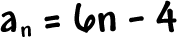Remember when I did that list with the a's to describe a sequence...And I told you this was the nth term?

That probably didn't mean much to you at the time.  Here's what we use this for:

The nth term is given by a formula.
We can use this formula to build the sequence.

Check it out:

Let's build the sequence whose nth term is given by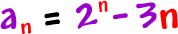See the n's in this guy?

If we let n = 1, we'll get the first term of the sequence: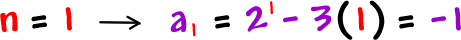If we let n = 2, we'll get the second term: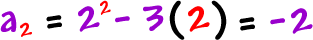If we let n = 3, we'll get the third term:and so on...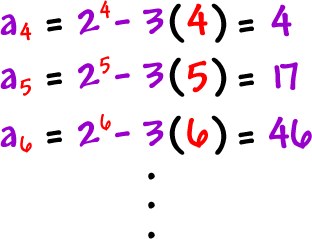So, our sequence isIt's easy!

When you're given a formula for, you stick in n = 1, then
n = 2, then 3, 4, and 5 to get the first five terms.

TRY IT:

Build the sequence (the first five terms) whose nth term is given by